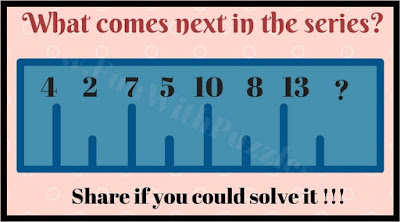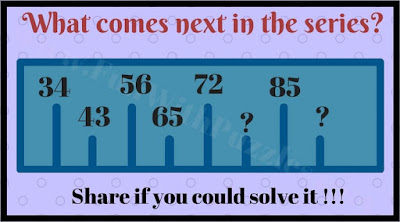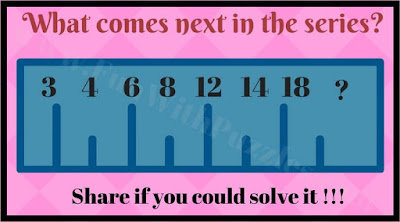## Friday, May 1, 2020

A few days back we have posted two math sequence. One was titled "What is Next Number In The Sequence?" in which one has to find the next number in the mathematical series. Another one was titled "Maths Number Sequence Puzzles with answers" in which there were nine numbers given in each of puzzle in three different rows. These numbers were related to each other mathematically or logical and one has to find the missing number in each puzzle. Both of these puzzles were very much liked by our visitors. Here is another set of mind-twisting math pattern series puzzles.
In each of these math series riddles, some numbers are given. These numbers follow some mathematical or logical sequence. One has to find this pattern which relates these numbers and has to find the missing numbers in the series.
Answers to these mind-twisting math series riddles are given at the end. Do post your answers with an explanation in the comment section.1. What will replace the question mark?2. What comes next in the series?3. Tricky math sequence pattern riddle4. Mind twisting math sequence puzzle5. Mind-blowing sequence picture puzzle

Answers of these puzzles are given as below

A1. 62

A2. 61 and 125

A3. 11

A4. 27 and 58

A5. 20

1.1.